# 15 Population simulations

## 15.1 Basic population simulation

In pharmacometrics, population simulations are integral parts of modeling projects. On one hand they are used to check the predictiveness of the model (i.e., visual predictive checks). On the other hand simulations of virtual population support decisions, e.g., to evaluate trial designs or new, proposed posologies.

In chapter Simulation of models, we discussed the simulation of one set of parameters in combination with one dosing regimen. We show in this chapter how to efficiently simulate populations, i.e., run model simulations for multiple sets of parameters and dosing regimens. While using the same function (sim_IQRmodel()) to execute simulations as for the simple simulations, we make use of the input argument eventTable as alternative to the dosingTable and parameters. The eventTable input needs to the an IQReventTable object combining individual sets of parameters with individual dosing and potentially containing further information needed for simulation.

To give a basic overview steps to perform population simulations we go through a basic example before the customization of the simulation setup is discussed:

### 15.1.1 Basic example

The first example makes use of a base 1-compartmental PK model for theophilline that was estimated based on 12 subjects recieving a single dose of 400 mg. To perform a simulation of a population, generally, we need to define the parameters and dosing for each individual respectively.

We load the individual estimates from the model results:

# Get individual estimated parameters
indivEstimates <- getIndivParameters_IQRnlmeProject(projectPath = "material/01-07-PopulationSimulation/ExTheophilline/NONMEM")
# Get names of the parameters
paraNames <- setdiff(names(indivEstimates), c("ID", "USUBJID"))
Table 8.1: Individual parameter estimates for theophilline PK
ID ka CL Vc USUBJID
1 0.0843322 2.71736 1.934910 Theo_1
2 0.0846147 2.58490 3.918930 Theo_10
3 0.0847180 4.14159 1.080320 Theo_11
4 0.0848563 2.83536 2.867530 Theo_12
5 0.0847785 3.61201 1.697320 Theo_2
6 0.0846263 3.56896 1.540070 Theo_3
7 0.0846547 3.34095 2.755240 Theo_4
8 0.0847636 2.91772 1.923560 Theo_5
9 0.0845443 4.51239 3.500380 Theo_6
10 0.0846042 3.74884 4.647100 Theo_7
11 0.0846161 3.86705 2.661180 Theo_8
12 0.0846867 4.07831 0.655227 Theo_9

We have to add the dosing information that is done using the same columns as for modeling datasets in the IQRdataGENERAL format or as used as inputs to define a dosing with IQRdosing(), i.e., by defining ID, TIME, ADM, AMT, TINF, RATE, ADDL, and II. As for modeling datasets or the IQRdosing() function, TIME, ADM and AMT are required while the others are optional and required depending on the type of dosing that is to be defined. For example, infusions need to have either the infusion time (TINF) ot the rate of infusion (RATE) defined whereas ADDL and II are superflous for single doses. As for this case all individuals receive a single dose of 400 mg theophilline at time = 0, we can simply add this information to the data frame with individual parameters:

# Add dosing information
eventData <- within(indivEstimates, {
TIME <- 0
AMT  <- 400
})

The IQReventTable is created based on the data frame eventData using the function IQReventTable() that requires to specify the names of the individual parameters that should be used in the simulation as the regression input.

# Create event table with dosing and individual parameter information
eventTable <- IQReventTable(eventData, regression = paraNames)
Table 8.2: Event table to simulate individual predictions of theophilline PK
ID TIME ADM AMT TINF ka CL Vc
1 0 1 400 1e-04 0.0843322 2.71736 1.934910
2 0 1 400 1e-04 0.0846147 2.58490 3.918930
3 0 1 400 1e-04 0.0847180 4.14159 1.080320
4 0 1 400 1e-04 0.0848563 2.83536 2.867530
5 0 1 400 1e-04 0.0847785 3.61201 1.697320
6 0 1 400 1e-04 0.0846263 3.56896 1.540070
7 0 1 400 1e-04 0.0846547 3.34095 2.755240
8 0 1 400 1e-04 0.0847636 2.91772 1.923560
9 0 1 400 1e-04 0.0845443 4.51239 3.500380
10 0 1 400 1e-04 0.0846042 3.74884 4.647100
11 0 1 400 1e-04 0.0846161 3.86705 2.661180
12 0 1 400 1e-04 0.0846867 4.07831 0.655227

Having the dosing and the parameters for all individuals combined in an IQReventTable object, we can use it for simulation:

# Get structural model from the NLME project
model <- IQRmodel("material/01-07-PopulationSimulation/ExTheophilline/NONMEM/model.txt")
# Load data for plotting
# Define simulation tims
simtime <- seq(min(data$TIME), max(data$TIME), length.out = 201)
# Simulate
simData <- sim_IQRmodel(model, simtime, eventTable = eventTable)
# Plot individual predictions and observations
ggplot(simData, aes(TIME, OUTPUT1)) + geom_line() + facet_wrap(~ID) + geom_point(data=data, aes(y=DV), shape = 1)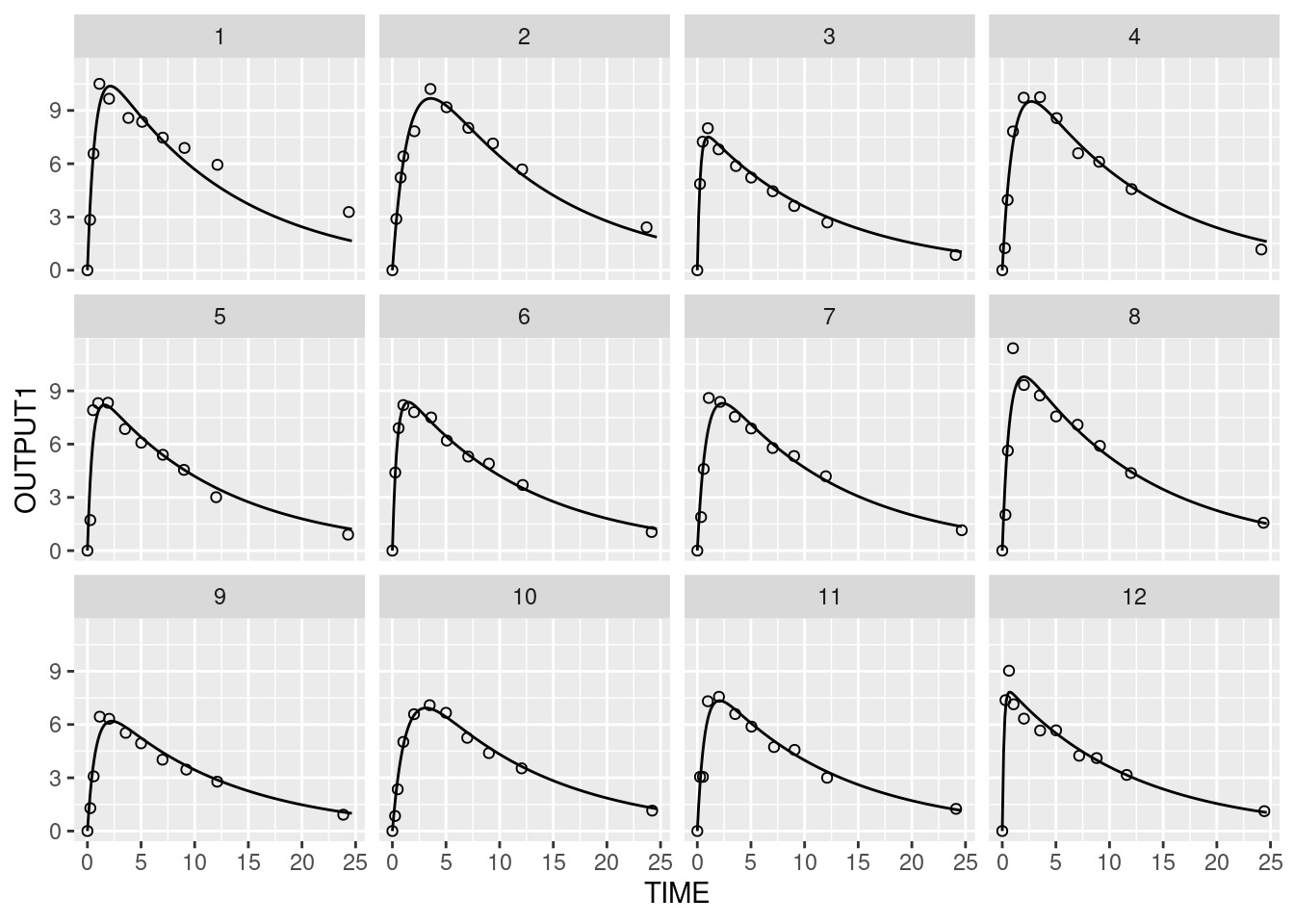### 15.1.2 Event table generation

#### Extract dosing from dataset

dataNLME    <- IQRloadCSVdata("./material/01-07-PopulationSimulation/Ex2cptModel/data.csv")
dosing <- extract_IQRdosing(dataNLME)
dosing <- extract_IQRdosing(dataNLME, FLAGunique = TRUE, TIMEcol = "TIME")
eventDataList <- lapply(dosing, function(x) create_IQReventData(x, indivEstimates))

#### Individual dosing

dosing1 <- IQRdosing(TIME = 0, ADM = 1, AMT = 100, ADDL = 2, II = 24)
dosing2 <- IQRdosing(TIME = 0, ADM = 2, AMT = 50, TINF = 10)

dosing <- list(dosing1, dosing1, dosing2, dosing2)

create_IQReventData(dosing, indivEstimates[1:4,])
## Warning: List of dosings unnamed. Individuals in indivData are match by order of
## occurence.
##   TIME ADM AMT  TINF ID         ka      CL      Vc USUBJID
## 1    0   1 100 1e-04  1 0.08433216 2.71736 1.93491  Theo_1
## 2   24   1 100 1e-04  1 0.08433216 2.71736 1.93491  Theo_1
## 3   48   1 100 1e-04  1 0.08433216 2.71736 1.93491  Theo_1
## 4    0   1 100 1e-04  2 0.08461466 2.58490 3.91893 Theo_10
## 5   24   1 100 1e-04  2 0.08461466 2.58490 3.91893 Theo_10
## 6   48   1 100 1e-04  2 0.08461466 2.58490 3.91893 Theo_10
## 7    0   2  50 1e+01  3 0.08471802 4.14159 1.08032 Theo_11
## 8    0   2  50 1e+01  4 0.08485631 2.83536 2.86753 Theo_12

#### Time-varying parameters

dosing <- IQRdosing(TIME = 0, ADM = 1, AMT = 100, ADDL = 3, II = 24)

indivParameters <- indivEstimates[1:2,]
indivParameters$ID = 1 indivParameters$TIME = c(0,10)

create_IQReventData(dosing, indivParameters)
##   TIME ADM AMT  TINF ID         ka      CL      Vc USUBJID
## 1    0   1 100 1e-04  1 0.08433216 2.71736 1.93491  Theo_1
## 2   10  NA  NA    NA  1 0.08461466 2.58490 3.91893 Theo_10
## 3   24   1 100 1e-04  1         NA      NA      NA    <NA>
## 4   48   1 100 1e-04  1         NA      NA      NA    <NA>
## 5   72   1 100 1e-04  1         NA      NA      NA    <NA>

### 15.1.3 Parameter sampling

Often, models are simulated for sets of parameters sampled from an NLME model. Virtual populations are simulated based on sampled individual parameters. Or, the prediction uncertainty for a typical subject is evaluated using simulations with parameters sampled from the unceratinty distribution.

The function sample_IQRnlmeProject() is used to generate various sets of sampled individual and/or population parameters. An important input argument to this function controlling the sampling is FLAG_SAMPLE as it determines whether uncertainty, IIV, and/or covariates are considered when creating the sets of model parameters.

FLAG_SAMPLE Sampling behavior
0 Use point estimates of population parameters (do not consider uncertainty) and sample Nsample individual patients based on these. Covariates considered if defined by user and used in model. Please note: population parameters do not take covariates into account!
1 Sample single set of population parameters from uncertainty distribution and sample Nsample individual patient parameters based on these. Covariates considered if defined by user and used in model. Please note: population parameters do not take covariates into account!
2 Sample Nsample sets of population parameters from uncertainty distribution. Do not sample from variability distribution and do not take into account covariates (even if user specified).
3 Use point estimates of population parameters (do not consider uncertainty) Return Nsamples sets of population parameters with covariates taken into account.
4 Sample single set of population parameters from uncertainty distribution Return Nsamples sets of population parameters with covariates taken into account.

Different use cases of sample_IQRnlmeProject() are illustrated in the following. The example model used is a 2-compartmental model that was build on single and multiple dose data and contains age, gender, and body weight as covariates:

# Example NLME project to illustrate parameter sampling
projectNLME <- as_IQRnlmeProject("material/01-07-PopulationSimulation/Ex2cptModel")
dataNLME    <- getData_IQRnlmeProject(projectNLME)
model <- IQRmodel("material/01-07-PopulationSimulation/Ex2cptModel/model.txt")

#### Virtual population

Individual parameters are sampled from an NLME project using the sample_IQRnlmeProject() function by specifying the path to the NLME project and specifying and the number of individual parameter to be returned.

# Number of individuals
Nind <- 100
# Sample individual parameters
sampleParameters <- sample_IQRnlmeProject(projectNLME, Nsamples = Nind)
##
## No continuous covariates for sampling have been defined but the model contains the following:
## AGE0 WT0
##
##
## No categorical covariates for sampling have been defined but the model contains the following:
## SEX
# Outputs
sampleParameters$FLAG_SAMPLE ##  0 sampleParameters$Nsamples
##  100
sampleParameters$popParamValues ## CL Vc ka Q1 Vp1 ## 28.6210094 31.0098010 0.2437317 22.8036843 2324.8062961 head(sampleParameters$indParamValues)
##         CL       Vc        ka       Q1       Vp1
## 1 27.77810 26.93113 0.1763787 16.92341 3055.5520
## 2 26.37272 23.67334 0.1958432 26.89455 1870.9154
## 3 30.01537 27.46824 0.1840414 32.15079  946.1897
## 4 17.54882 20.20632 0.4441324 22.10915 2928.7995
## 5 18.72291 16.60976 0.2170710 56.31248 1875.8135
## 6 47.58107 39.68856 0.2072612 17.27045 1692.6324

The function returns information about the sampling (FLAG_SAMPLE and Nsamples), population parameters (popParamValues) and individual parameters (indParamValues). For default, the function does not consider uncertainty (FLAG_SAMPLE = 0), thus the virtual population is based on the point estimates of the model parameters.

To sample with considering covariates, the input argument covariates is used which can a data frame or matrix with individual information per row and named columns containing the covariate values. Note that the function return a data frame of individual parameter including also the subject ID which is later needed to create an event table for simulation.

# Define covariates for individuals
covariates <- data.frame(
ID = 1:Nind,
SEX = sample(c(1,2), Nind, replace = TRUE),
AGE0 = runif(Nind, min=20, max = 65),
WT0 = runif(Nind, min = 45, max = 100)
)
# Sample individual parameters while considering covariate information
indivParameters <- sample_IQRnlmeProject(projectNLME, covariates = covariates, FLAGid = TRUE)$indParamValues # Visualize covariates dependency of parameters x <- merge(covariates, indivParameters, by = "ID") p1 <- ggplot(x, aes(WT0, ka)) + geom_point() + geom_smooth() + scale_y_log10() p2 <- ggplot(x, aes(AGE0, ka)) + geom_point() + geom_smooth() + scale_y_log10() p3 <- ggplot(x, aes(as.factor(SEX), CL)) + geom_boxplot() + scale_y_log10() gridExtra::grid.arrange(p1,p2,p3, nrow = 1)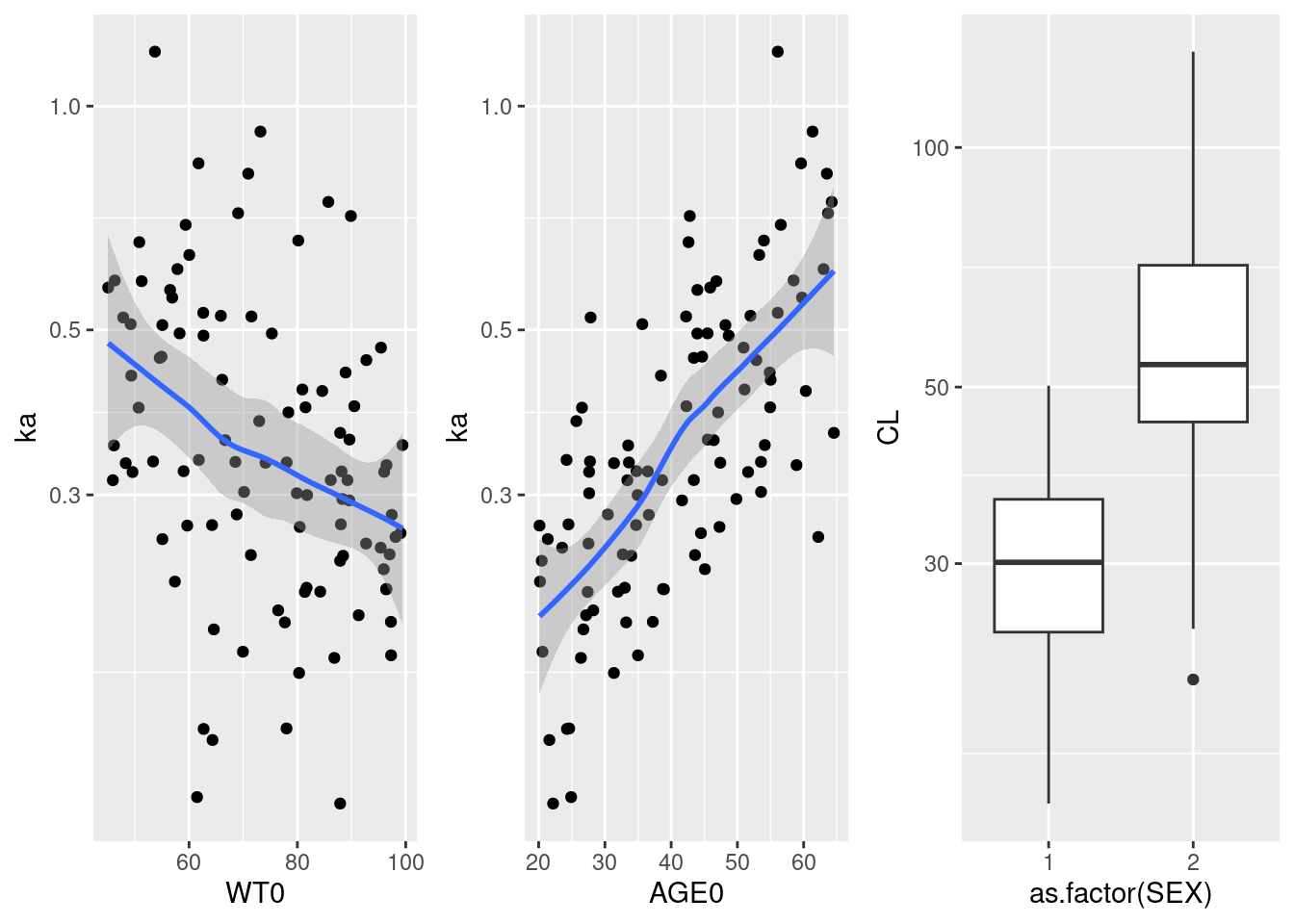#### Virtual populations considering estimation uncertainty To simulate population while also accounting for parameter estimation uncertainty, we need to set FLAG_SAMPLE = 1. Several populations are created for which new population-level parameters (fixed effects and distribution of IIVs) are sampled from the parameter covariance matrix: # Number of populations Npop <- 100 # Sample individual parameters for each population sampleParametersPop <- lapply(1:Npop, function(k) sample_IQRnlmeProject(projectNLME, FLAG_SAMPLE = 1, covariates = covariates, FLAGid = TRUE) ) names(sampleParametersPop) <- 1:Npop # Collect individual parameters into data frame indivParametersPop <- plyr::ldply(sampleParametersPop, function(x) x$indParamValues, .id = "IDpop")
indivParametersPop$IDpop <- as.numeric(indivParametersPop$IDpop)
indivParametersPop$ID <- indivParametersPop$ID + Nind*10 * (indivParametersPop$IDpop-1) # Collect population parameters into data frame popParametersPop <- plyr::ldply(sampleParametersPop, function(x) x$popParamValues, .id = "IDpop")
popParametersPop$IDpop <- as.numeric(popParametersPop$IDpop)
ggplot(indivParametersPop, aes(IDpop, CL)) + geom_point(shape=1, alpha= 0.3) +
geom_point(data=popParametersPop, color = "firebrick") + scale_y_log10()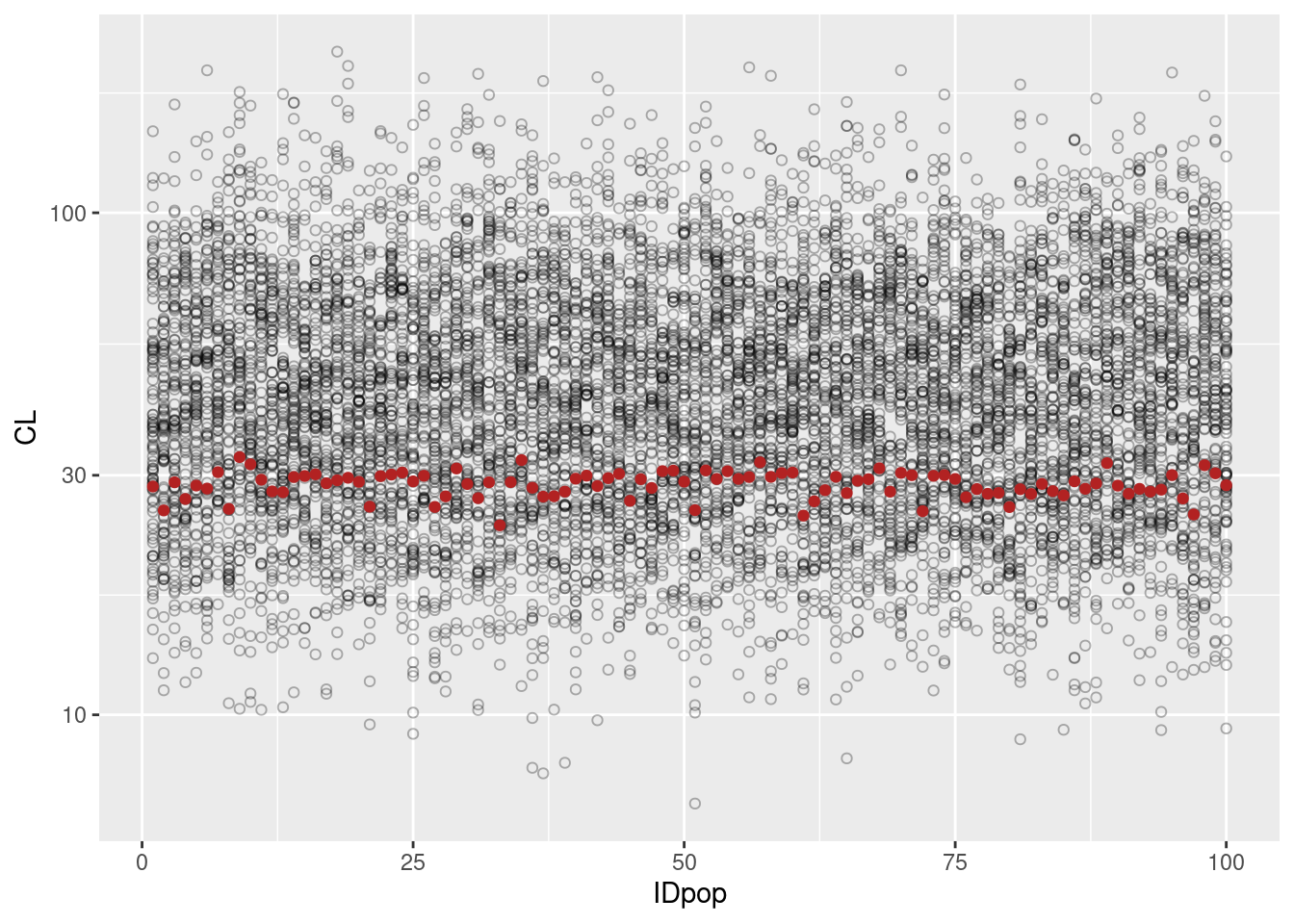#### Prediction uncertainty for typical subject

The prediction uncertainty is assessed when sampling only from the uncertainty of the estimated parameter distribution.

# Sample parameters
popParameters <- sample_IQRnlmeProject(projectNLME, Nsamples = 100, FLAG_SAMPLE = 2, FLAGid = TRUE)$popParamValues # Add dosing eventData <- create_IQReventData(IQRdosing(TIME = 0, ADM = 1, AMT = 100), popParameters) # Create event table eventTable <- IQReventTable(eventData, regression = setdiff(names(popParameters),"ID")) # Simulate simUncertainty <- sim_IQRmodel(model, simtime = seq(0.2,80, by = 0.2), eventTable = eventTable, FLAGoutputsOnly = TRUE) # Plot predictions and confidence interval ggplot(simUncertainty, aes(TIME,OUTPUT1)) + scale_y_log10()+ stat_summary(fun.data = median_hilow, geom = "ribbon", alpha = 0.3) + stat_summary(fun.data = median_hilow, geom = "line") + ggtitle("Simulation of reference subject with 95%-CI")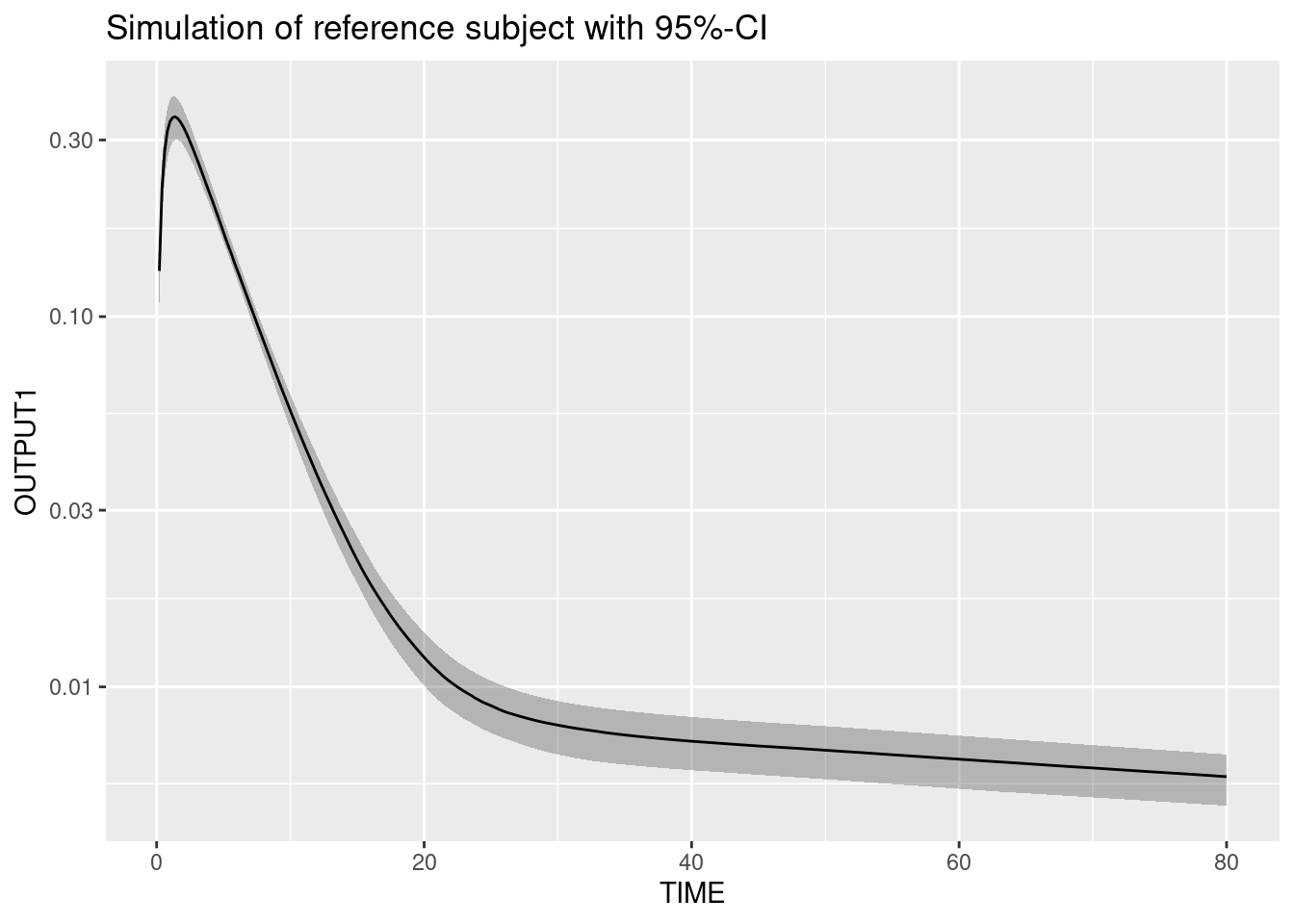#### Prediction differences due to covariates Prediction differences exclusively due to covariate characteristics can be assessed using the FLAG_SAMPLE = 3. Here, we show differences betwenn the male and the female subpopulations. # Sample parameters popParameters <- sample_IQRnlmeProject(projectNLME, covariates = covariates, FLAG_SAMPLE = 3, FLAGid = TRUE)$popParamValues
eventData <- create_IQReventData(IQRdosing(TIME = 0, ADM = 1, AMT = 100), popParameters)
# Create event table
eventTable <- IQReventTable(eventData, regression = setdiff(names(popParameters),"ID"))
# Simulate
simCovDifference <- sim_IQRmodel(model, simtime = seq(0.2,80, by = 0.2), eventTable = eventTable, FLAGoutputsOnly = TRUE)
# Plot predictions and confidence interval
simCovDifference <- merge(simCovDifference, covariates, by = "ID")
simCovDifference$SEX <- factor(simCovDifference$SEX, levels = c(1,2), labels = c("male", "female"))
ggplot(simCovDifference, aes(TIME,OUTPUT1, color = SEX, fill = SEX, group = SEX)) +
stat_summary(fun.data = median_hilow, geom = "ribbon", alpha = 0.3) +
stat_summary(fun.data = median_hilow, geom = "line") +
scale_y_log10() + scale_color_manual(values = c("darkorange", "olivedrab")) + scale_fill_manual(values = c("darkorange", "olivedrab")) +
ggtitle("Differences for female and male subpopulation considering covariates, but no IIV")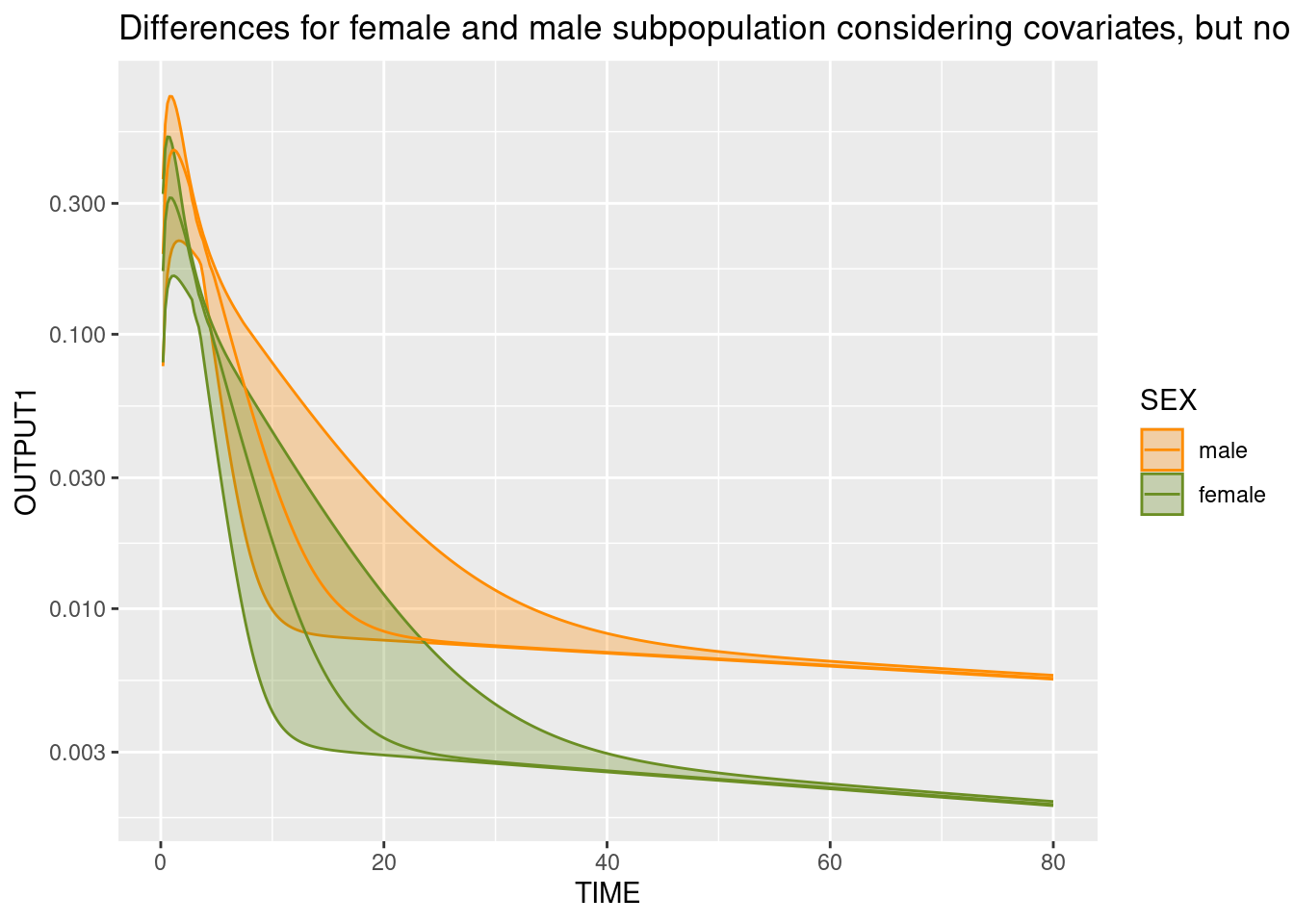#### Predictions for covariate differences with uncertainty

Prediction differences exclusively due to covariate characteristics can be assessed using the FLAG_SAMPLE = 3. Here, we show differences betwenn the male and the female subpopulations.

# Sample population parameters for each population
sampleParametersPop <- lapply(1:Npop, function(k)
sample_IQRnlmeProject(projectNLME, FLAG_SAMPLE = 4, covariates = covariates, FLAGid = TRUE)
)
names(sampleParametersPop) <- 1:Npop
# Collect population parameters into data frame
popParametersPop <- plyr::ldply(sampleParametersPop, function(x) x$popParamValues, .id = "IDpop") popParametersPop$IDpop <- as.numeric(indivParametersPop$IDpop) popParametersPop$ID <- popParametersPop$ID + Nind*10 * (popParametersPop$IDpop-1)
eventData <- create_IQReventData(IQRdosing(TIME = 0, ADM = 1, AMT = 100), popParametersPop)
# Create event table
eventTable <- IQReventTable(eventData, regression = setdiff(names(popParameters),c("ID", "IDpop")))
# Simulate
simCovDifference <- sim_IQRmodel(model, simtime = seq(0.2,80, by = 0.2), eventTable = eventTable, FLAGoutputsOnly = TRUE)
# Calculate median prediction per male/female subpopulation
simCovDifference <- merge(simCovDifference, popParametersPop[,c("ID", "IDpop")])
simCovDifference$ID <- simCovDifference$ID %% 1000
simCovDifference <- merge(simCovDifference, covariates, by = "ID")
simCovDifference$SEX <- factor(simCovDifference$SEX, levels = c(1,2), labels = c("male", "female"))

simCovDifferenceMedian <- plyr::ddply(simCovDifference, ~IDpop+TIME+SEX, function(x) c(Median = median(x\$OUTPUT1)))

ggplot(simCovDifferenceMedian, aes(TIME, Median, color = SEX, fill = SEX, group = SEX)) +
stat_summary(fun.data = median_hilow, geom = "ribbon", alpha = 0.3) +
stat_summary(fun.data = median_hilow, geom = "line") +
scale_y_log10() + scale_color_manual(values = c("darkorange", "olivedrab")) + scale_fill_manual(values = c("darkorange", "olivedrab")) +
ggtitle("Difference between median of female and male subpopulations with 95-CI")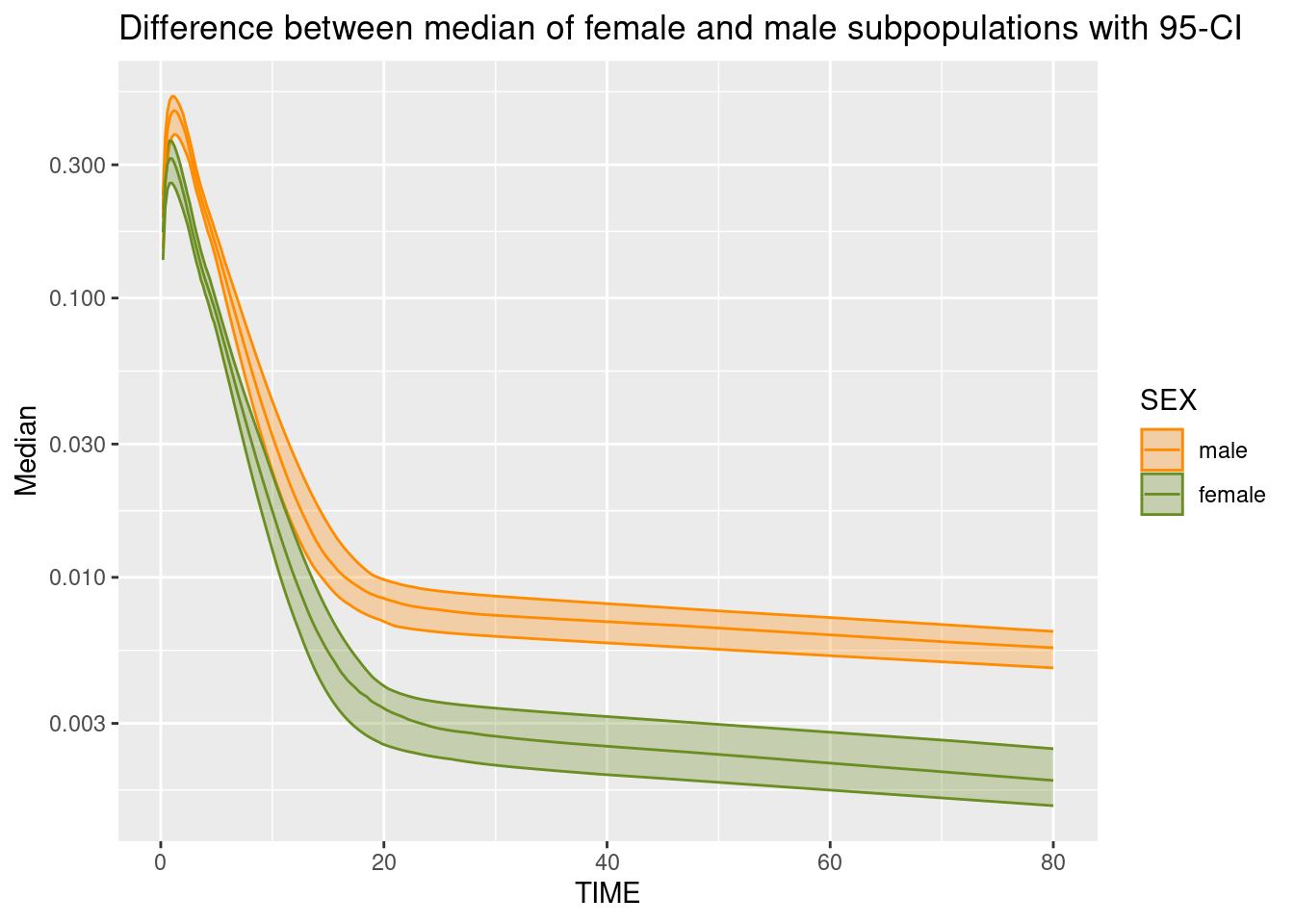## 15.2 Clinical trial simulation

### 15.2.1 Parallel design example

Example will be added soon.

### 15.2.2 Adaptive design example

Example will be added soon.